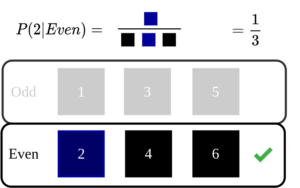## An Intuitive Explanation of Naive Bayes Classifier

Introduction In this post, let’s take a look at the intuition behind Naive Bayes Classifier used in machine learning. Naive Bayes classifier is one of the basic algorithms often encountered in machine learning applications. If linear regression was based on concepts from linear algebra and calculus, naive Bayes classifier mostly backed up by probability theory. …